#INFOSWMM

# Objective Functions in the Genetic Algorithm of InfoSWMM and InfoSWMM SA

Objective Functions in the Genetic Algorithm of InfoSWMM and InfoSWMM SA

The objective of the optimal calibration problem is to minimize the numerical discrepancy between the observed and predicted values of link flow, link depth, and/or link velocity at various locations in the system. Any of the following five different mathematical objective functions can be used in InfoSWMM && InfoSWMM SA Calibrator.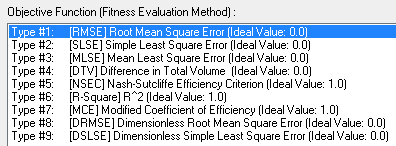Root Mean Square Error (RMSE)In the ideal condition when corresponding observed and simulated values exactly match, value of RMSE will be zero.Simple Least Square Error (SLSE)This performance evaluation function could assume values ranging from zero (best fit) to ∞ (poor fit), and it tends to favor large errors and large (i.e., peak) flows.Mean Simple Least Square Error (MSLSE)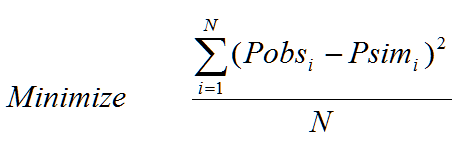This performance evaluation function could assume values ranging from zero (best fit) to ∞ (poor fit), and it tends to favor large errors and large (i.e., peak) flows.Difference in Total VolumeDifference in total volume could range from -∞ (poor performance) to ∞ (poor performance), the ideal value being zero (i.e., exact match between total simulated volume and total observed volume).Nash-Sutcliffe Efficiency Criterion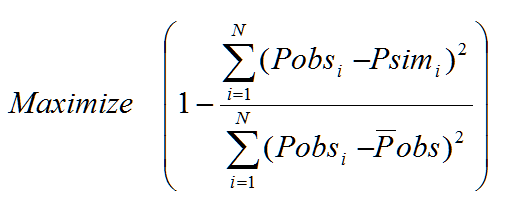This efficiency criterion could assume values from -∞ (poor performance) to 1 (perfect model).R-Square (R2)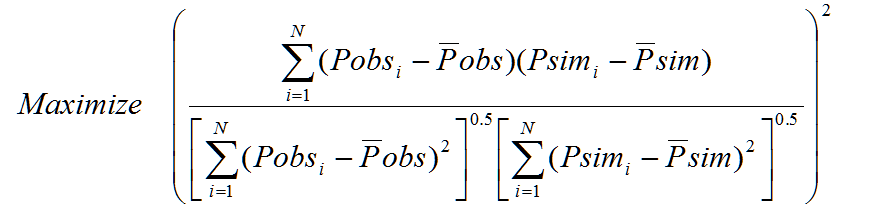R2 value varies from zero (indicates worst fit) to unity (indicates perfect fit).Modified Coefficient of EfficiencyThe modified coefficient of efficiency could assume values from -∞ (poor performance) to 1 (perfect model).Dimensionless Root Mean Square Error (DRMSE)In the ideal condition when corresponding observed andDimensionless Simple Least Square Error (DSLSE)

Simulated values exactly match, value of DRMSE will be zero.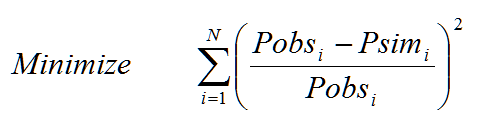This performance evaluation function could assume values ranging from zero (best fit) to ∞ (poor fit), and it tends to be independent of length of records and it favor large errors and large (i.e., peak) flows.

where N designates the total number of measurements available, Pobsi represents the observed measurement values at time i; Psimi is the model simulated values at time i;Pobs is mean of the measured values; Psim is the mean of the simulated values.

It is believed that complete assessment of model performance should include at least one relative error measure (e.g., modified coefficient of efficiency) and at least one absolute error measure (e.g., root mean square error) with additional supporting information (e.g., a comparison between the observed and simulated mean and standard deviation)2.

The number of field measurements defining the objective function must be greater than or equal to the number of calibration variables. It is expected that the accuracy of the model calibration would be increased by the use of a large number of field measurements. The decision variables could be any one or more of about fifty nfoSWMM && InfoSWMM SA parameters. These decision variables are automatically adjusted to minimize the objective function selected while satisfying a set of implicit and explicit constraints.

# Genetic Algorithms

Genetic algorithms (GA) are an adaptation procedure based on the mechanics of natural genetics and natural selection1. They are designed to perform search procedures of an artificial system by emulating the evolution process (Darwin’s evolution principle) observed in nature and biological organisms. The evolution process is based on the preferential survival and reproduction of the fittest member of a population with direct inheritance of genetic information from parents to offspring and the occasional mutation of genes. The principal advantages of genetic algorithms are their ability to converge expeditiously on an optimal or near-optimal solution without having to analyze all possible solutions available and without requiring derivatives or other auxiliary knowledge.

2.1 OVERVIEW OF GENETIC ALGORITHMS

Genetic algorithms are fundamentally different from traditional optimization methods in terms of the search process. While traditional routines track only a single pathway to the optimal solution, genetic algorithms search from an entire population of possible solutions (individuals). In addition, genetic algorithms use randomized and localized operators as opposed to deterministic rules. Each individual in the population is represented by either a string or a set of real numbers encoding one possible solution. The performance of each individual in the population is measured by its fitness (goodness), which quantifies the degree of optimality of the solution. Based on their fitness values, individuals are selected for reproduction of the next generation. Each new generation maintains its original size. The selected individuals reproduce their offspring by mimicking gene operations of crossover and mutation. After a number of generations, the population is expected to evolve artificially, and the optimal or near optimal solution is ultimately reached.

2.2 COMPONENTS OF GENETIC ALGORITHMS

Standard genetic algorithms involve three basic functions: selection, crossover, and mutation. Each function is briefly described below.

Selection – Individuals in a population are selected for reproduction according to their fitness values. In biology, fitness is the number of offspring that survive to reproduction. Given a population consisting of individuals identified by their chromosomes, selecting two chromosomes to represent parents to reproduce offspring is guided by a probability rule that the higher the fitness an individual has, the more likely the individual will survive. There are many selection methods available including weighted roulette wheel, sorting schemes, proportionate reproduction, and tournament selection.

Crossover - Selected parents reproduce the offspring by performing a crossover operation on the chromosomes (cut and splice pieces of one parent to those of another). In nature, crossover implies two parents exchange parts of their corresponding chromosomes. In genetic algorithms, a crossover operation makes two strings swap their partial strings. Since more fit individuals have a higher probability of producing offspring than less fit ones, the new population will possess, on average, an improved fitness level. The basic crossover is a one-point crossover. Two selected strings create two offspring strings by swapping the partial strings, which is cut by one randomly sampled breakpoint along the chromosome. The one-point crossover can easily be extended to k-point crossover. It randomly samples k breakpoints on chromosomes and then exchanges every second corresponding segments of two parent strings.

Mutation - Mutation is an insurance policy against lost genes. It works on the level of string genes by randomly altering gene value. With small probability, it randomly selects one gene on a chromosome then replaces the gene by a randomly selected value. The operation is designed to prevent GA from premature termination namely converging to a solution too early.

Elitism - The selection and crossover operators will tend to ensure that the best genetic material and the components of the fittest chromosomes will be carried forward to the next generation. However, the probabilistic nature of these operators implies that this will not always be the case. An elitist strategy is therefore required to ensure that the best genetic material will not be lost by chance. This is accomplished by always carrying forward the best chromosome from one generation to the next.

The flowchart below illustrates the optimal calibration process.